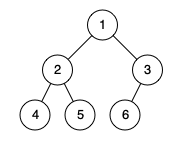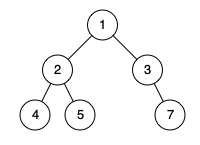Formatted question description: https://leetcode.ca/all/958.html

# 958. Check Completeness of a Binary Tree (Medium)

Given a binary tree, determine if it is a complete binary tree.

Definition of a complete binary tree from Wikipedia:
In a complete binary tree every level, except possibly the last, is completely filled, and all nodes in the last level are as far left as possible. It can have between 1 and 2h nodes inclusive at the last level h.

Example 1:Input: [1,2,3,4,5,6]
Output: true
Explanation: Every level before the last is full (ie. levels with node-values {1} and {2, 3}), and all nodes in the last level ({4, 5, 6}) are as far left as possible.


Example 2:Input: [1,2,3,4,5,null,7]
Output: false
Explanation: The node with value 7 isn't as far left as possible.


Note:

1. The tree will have between 1 and 100 nodes.

Companies:

Related Topics:
Tree

## Solution 1.

Use level order traversal. After visiting a node that has at least one null child, all the subsequent nodes must be leaf nodes.

// OJ: https://leetcode.com/problems/check-completeness-of-a-binary-tree/

// Time: O(N)
// Space: O(N)
class Solution {
public:
bool isCompleteTree(TreeNode* root) {
if (!root) return true;
queue<TreeNode*> q;
q.push(root);
bool onlyLeafNow = false;
while (q.size()) {
int cnt = q.size();
while (cnt--) {
root = q.front();
q.pop();
if (!root->left && root->right) return false;
if (onlyLeafNow && (root->left || root->right)) return false;
if (root->left) q.push(root->left);
if (root->right) q.push(root->right);
if (!root->left || !root->right) onlyLeafNow = true;
}
}
return true;
}
};


Java

/**
* Definition for a binary tree node.
* public class TreeNode {
*     int val;
*     TreeNode left;
*     TreeNode right;
*     TreeNode(int x) { val = x; }
* }
*/
class Solution {
public boolean isCompleteTree(TreeNode root) {
if (root == null)
return true;
Queue<TreeNode> queue = new LinkedList<TreeNode>();
queue.offer(root);
int levelNodes = 1;
while (!queue.isEmpty()) {
int size = queue.size();
if (size == levelNodes) {
boolean flag = true;
for (int i = 0; i < size; i++) {
TreeNode node = queue.poll();
TreeNode left = node.left, right = node.right;
if (left == null && right != null)
return false;
if (left != null)
queue.offer(left);
if (right != null)
queue.offer(right);
if (!flag && (left != null || right != null))
return false;
if (flag && (left == null || right == null))
flag = false;
}
} else {
for (int i = 0; i < size; i++) {
TreeNode node = queue.poll();
if (node.left != null || node.right != null)
return false;
}
}
levelNodes <<= 1;
}
return true;
}
}